Next: Methods to Increase Efficiency Up: Numerical Tasks in Molecular Previous: Energy Function

## Integration Methods

The integration method of the Newtonian equations of motion employed by our program is the Verlet algorithm . This method determines the positions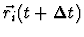, of atoms i at the instant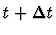according to the formula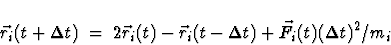where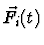stands for the sum of all forces acting on the i-th atom at time t, i.e.,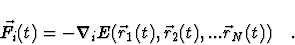While integrating the Newtonian equations of motion computer time is spent mainly on evaluation of the two-particle interactions, i.e., of interactions originating from the Coulomb potential EEl and the van der Waals energy EvdW. The programs CHARMM and X-PLOR avoid the prohibitive computational effort of an exact evaluation by allowing a cut-off for these interactions; this assumes that these interactions do not contribute much to the dynamics for pairs of atoms separated beyond a certain distance. (See below.)

We have not introduced such a criterion into our program. Rather than providing a cut-off option we introduced an option which makes it possible to evaluate the Coulomb interaction in a hierarchical way such that, according to a hierarchy of inter-particle distances, Coulomb forces are updated with different frequencies. Such an algorithm has been suggested in  and is described in [23,21] and in Section 6.2.1 of this documentation. An alternative method for an efficient evaluation of Coulomb forces, the Fast Multipole Algorithm, has been developed by Greengard and Rokhlin [19,20,29] and is used in EGO simultanously for rapid evaluation of long range forces. The combination of both algorithms we termed FAMUSAMM.

Calculation of van der Waals and Coulomb forces is the most time consuming task in molecular dynamics calculations. The forces connected with the chemical bonds of biopolymers are determined much more rapidly during program execution. Because of the essentially linear arrangement of biopolymers the respective calculations can be readily ordered in a linear fashion and, therefore, a strategy for parallel computation of forces connected with chemical bonds is straightforward. Hence, we will not explain how these interactions are evaluated. We would like to close this section with a brief description of the input-output requirements of our molecular dynamics program. As input the program needs a file of force parameters, a PDB file of atomic coordinates in protein data bank format, and a PSF protein structure file with definitions of bonds, dihedral and improper angles, etc. The file formats are identical to those of CHARMM and X-PLOR. As output the program delivers atomic coordinates in an internal format which may be converted on the host computer into any format for analysis of trajectory properties by CHARMM, X-PLOR or other programs.Next: Methods to Increase Efficiency Up: Numerical Tasks in Molecular Previous: Energy Function
Helmut Heller
2000-04-19